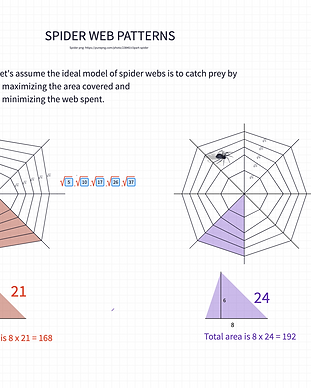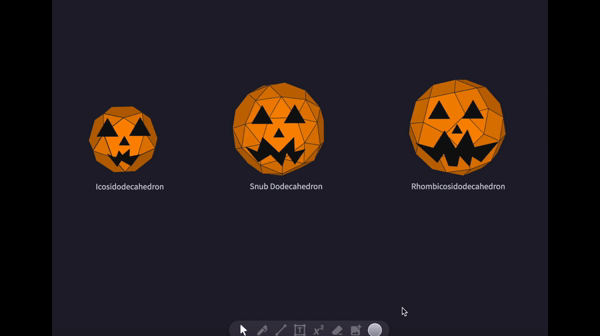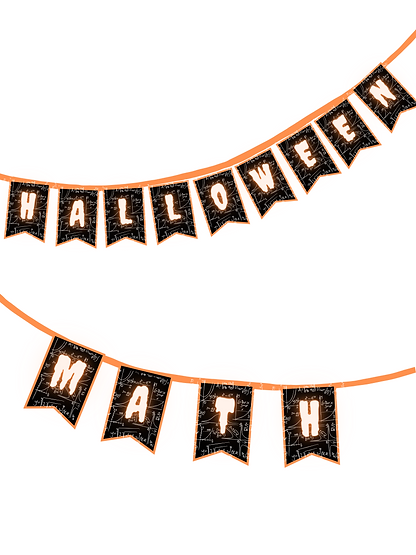# Math Fan Content

##### Halloween Math

Spider Webs

For some of us, spiders are highly frightening, which is why they are an essential component of the Halloween notion.

Do you wonder what are good mathematical models for spider webs?

We may start by thinking about why spiders weave their webs in similar forms. It appears that there could be extremely practical reasons for it, such as the size and strength of the web or the amount of energy required to construct one.

Let's assume the ideal model of spider webs is to catch prey by

- maximizing the area covered and

- minimizing the web spent.

Ask students to construct different webs to calculate their area and perimeter using the octagonal templates. Here is a sample Polypad file.

Creating Spider Webs using String Art

There are different ways to integrate a string art project into math lessons.

You may start from scratch and let students use a ruler and compass to create equal intervals on the lines. Or you may use the templates below to create some beautiful art pieces.

Here is the Polypad lesson plan of a task to investigate the maximum number of points, and regions formed by intersecting lines. Use the dark mode to create your spider web designs.

Once you create the shapes, you may realize they belong to different functions. Here is the x^2/3 + y​^2/3 = c^2/3

This particular curve is called an astroid.

Can you find the length of the curve or the area enclosed by the curve? Do this measure depend on the number of points on the initial lines?You may also use Archimedean solids Activity.

Explore the properties of Archimedean solids with students while turning them into pumpkins.

Archimedean solids are a special group of 13 semi-regular polyhedrons. An Archimedean solid has faces of two or more different types of regular polygons (their sides are all of equal lengths), such as squares, pentagons, hexagons, octagons, decagons, and triangles.Witch Hat Drawing Contest with Desmos

One of the best activities of all time is trying to re-create a shape & picture using graphs. Students explore many different properties of graphs while working on their projects.

Here is an example;and here are more Halloween surprises ..